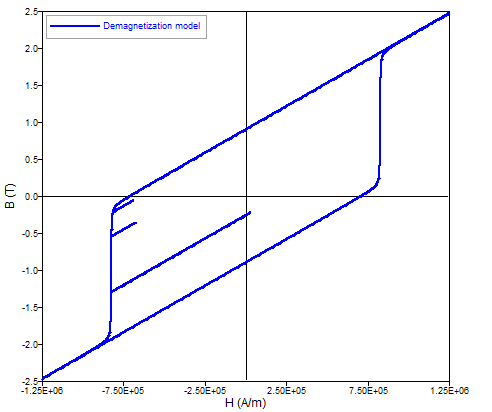# Magnet (unidirectional): demagnetization curve (Hc, Br)

## Presentation

This model ( Nonlinear magnet described by Hc and the Br module ) defines a nonlinear B(H) dependence with taking into account of demagnetization, wherever the curve knee is.

Main characteristics:

• the mathematical model and the direction of magnetization are dissociated
• a single material for description of several regions

## Mathematical model

In the direction of magnetization the model is a combination of a straight line and an arc tangent curve.

The corresponding mathematical formula is written:with:where:

• μ0 is the permeability of vacuum, μ0 = 4 π 10-7 (H/m)
• μrmax is the maximal relative permeability of material
• Br is the remanent flux density (T)
• Js is the saturation magnetization (T)

The shape of the B(H) dependence in the direction of magnetization is given in the opposite figure.In transversal directions one can write:

B(H)= μ0μr⊥H

where μr⊥ is the transverse relative permeability

## Direction of magnetization

The various possibilities provided to the user are the same ones as those presented in § Magnet (unidirectional): linear approximation.

## Demagnetization during solving

With this non-linear model, it is now possible consider the demagnetization during solving (i.e. the degradation of a magnet's remanent magnetic flux density due to its interaction with a demagnetizing field) by checking the specific option that is provided during the material creation. This demagnetization model is based on a static Preisach approach, and may be selected during the description of the B(H) property of this type of material. The following remarks apply:

• Available for 2D and 3D in transient magnetic application
• In projects containing a magnet material with this option active, it is advisable to set the application to perform an initialization through a static calculation (Application > Transient initialization).
• This model does not take in account temperature variations.To use this model in a solved project :

• Destroy the results;
• Go in Application > Transient initialization and select : Initialization by static calculation;
• Create a new material Nonlinear magnet described by Hc and Br module
• Check the option Taking in account demagnetization during solving
• Assign the material to the regions representing the magnets in the project;
• Go to Physics > Face regions (in 2D) or Volume regions (in 3D) > Orient material for face / volume regions to inform the direction of magnetization in the magnet
• Run the scenario.

To display the current remanent magnetic flux density of a magnet at a given time step:

• Select the desired time step;
• Create a new Arrows plot, then select the magnet as support.
• Then, in the formula editor, click on button Br Magnet (available in the Magnetic Volume region tab) or type BrMagnet (without a space) in the formula field.
Note: In the formula editor, a related button Br is available and the user could also type the corresponding formula Br in the formula field. Remark that these recover the original remanent magnetic flux density stored in the material description, and not the remanent magnetic flux density modified by demagnetization effects at a given time step represented by BrMagnet.

## Example of results

Let's consider the surface permanent magnet motor below, which was represented in a Flux 3D project: For the control angle values ψ = [0 ; π/6; π/4 ; π/3] , we can see that there is a local effect on the remanent flux density, but the repartition depends ψ, as shown in the picture below:
This localized effect modifies the machine's performance (e.g., torque and back EMF, as illustrated in the picture below).